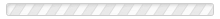Search:
(clear)
Results: 11
• ### m

• The magnetic moment is the strength of the response of a piece of metal to a magnetic field. It is a measure of the degree(...) Read More
• The magnetic moment of an electron is symbolized as g and is called the “g-factor.” The magnetic moment is the degree to(...) Read More
• Pi and e are examples of mathematical constants. A mathematical constant has the same value no matter which mathematical(...) Read More
• (Also called “observation”). Measurement is of considerable consequence in the quantum world. At least in some(...) Read More
• Understanding the Measurement Problem requires understanding the unique role of measurement in quantum mechanics. The entry(...) Read More
• A metal is a kind of matter, usually derived from rocks found in nature. Examples of metals include iron, aluminum, and(...) Read More
• A minimal theory in physics is a theory that accounts for known phenomena with the simplest approach conceivable. Of course,(...) Read More
• “Moment,” as used in physics, means the amount of a force that rotates the object. The term “moment” is used in regard to a(...) Read More
• Moment of force is also called torque. “Moment,” as used in physics, means the amount of force experienced by an object due(...) Read More
• In classical physics, moment of momentum is the momentum or oomph which an object has as it rotates about an axis. It is the(...) Read More
• (Abbreviated p. Momentum is the oomph something has due to having mass that’s in motion. Let’s say you just happen to be(...) Read More